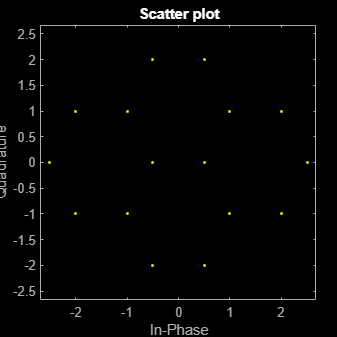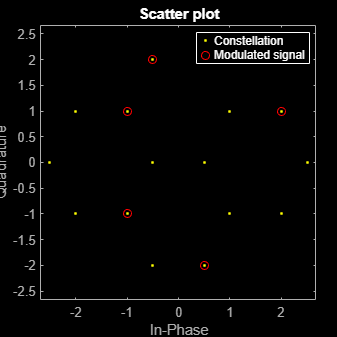# genqamdemod

General quadrature amplitude demodulation

## Syntax

``z = genqamdemod(y,const)``

## Description

example

````z = genqamdemod(y,const)` demodulates the complex envelope, `y`, of a quadrature amplitude modulated signal using the signal mapping specified in `const`.```

## Examples

collapse all

Create the points that describe a hexagonal constellation.

```inphase = [1/2 1 1 1/2 1/2 2 2 5/2]; quadr = [0 1 -1 2 -2 1 -1 0]; inphase = [inphase;-inphase]; inphase = inphase(:); quadr = [quadr;quadr]; quadr = quadr(:); const = inphase + 1i*quadr;```

Plot the constellation.

`h = scatterplot(const);`Generate input data symbols. Modulate the symbols using this constellation.

```x = [3 8 5 10 7]; y = genqammod(x,const);```

Demodulate the modulated signal, `y`.

`z = genqamdemod(y,const);`

Plot the modulated signal in same figure.

```hold on; scatterplot(y,1,0,'ro',h); legend('Constellation','Modulated signal');```Determine the number of symbol errors between the demodulated data to the original sequence.

`numErrs = symerr(x,z)`
```numErrs = 0 ```

## Input Arguments

collapse all

Complex envelope, specified as a scalar, vector, matrix, or 3-D array of numeric values. If `y` is a matrix with multiple rows, the function processes the rows independently.

Signal mapping, specified as a complex vector.

Data Types: `double` | `single`
Complex Number Support: Yes

## Output Arguments

collapse all

Message signal, returned as a scalar, vector, matrix, or 3-D array of numeric values. The message signal consists of integers between 0 and `length`(`const`)–1. The datatype of `z` is the same as the data type of input `x`.

Data Types: `double` | `single`

## Version History

Introduced before R2006a